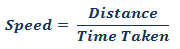1. Worksheets>
2. Math>
3. Solve>
4. Speed>
5. Distance and Time

# How to find the speed using distance travelled and time taken

Use this simple formula to calculate the speed:Solved Example 1:

Distance = 100 km and Time = 5 hr. Calculate speed.

Solution:

Using formula,Try yourself: Practice Sheet 1

Solved Example 2:

Distance = 127 miles and Time = 3 hr. Find the speed. (Round the answers to 2 decimal places)

Solution:

Using formula,Here 42.3333... is rounded to 2 decimal places.

Try yourself: Practice Sheet 2

Things To Know:

Unit of speed will be calculated based on unit of distance and time. For example, if the distances are in meters and the time in seconds, then the unit of speed will be meter per second (m/sec). There are certain standard form in calculating units. We do not usually calculate speed in km/sec or miles/sec. Standard units used in practical life are km/hr, m/sec and miles/hr.